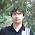## Sunday, January 6, 2019

### And the best problem of 2018 is...

According to the vote, the best problem of 2018 is the Open Cup problem about rolling a ball in a maze while collecting all targets in some order, by jh05013, with solution in this post. My vote also went to this problem.

Here's its statement in an abridged form once again: you are given a 50x50 grid where some cells are empty, and some are walls. There are also walls surrounding the grid. Some empty cells are marked as targets, and there's also a ball in one of the empty cells. You can move the ball in each of the four cardinal directions, but once it starts rolling, it rolls in the same direction until it hits the wall, at which point you can pick a new direction, and so on. Can you roll the ball in such a way that it passes through all targets in some order?

Congratulations to jh05013, to the Open Cup, and thanks to all problemsetters of 2018!

More precisely, since there were only 91 people voting, I'd say we're about 60% confident that the best problem of 2018 is that one :) Here's the Colab where I try estimate that confidence. Of course that number is meaningless without explaining the assumed underlying model yada yada, but I hope it's good enough for a ballpark estimate. If people who actually, unlike myself, understand how statistics and polling work are reading this: is that a good way to get confidence numbers for a poll? What is a better way?

Thanks for reading, and check back later today for this week's summary!

1.Where did you take this photo?

1.https://en.wikipedia.org/wiki/Sch%C3%B6llenen_Gorge

2.Cool, thanks a lot! :)

2.60% is a great ballpark! The Colab essentially calculates "Assuming this result as the true population distribution, what is the probability that a choice will win on 91 randomly selected votes?"

The number you actually want is roughly the converse: "If a randomly-selected population distribution produced this result in 91 votes, what is the probability of a choice being the true population max?" If you choose a uniformly random population distribution, the results are quite similar although the smaller choices are given significantly more mass -- 58% chance for problem 4 compared to 60% in your model, but 0.61% chance for problem 5 compared to your 0.34%.

1.Thanks! The reason I did not choose that path is that I could not explain to myself what is a uniformly random population distribution. Is it the one where we pick n-1 weights such that their sum is less than 1 and probability density is constant everywhere, and pick the n-th weight as one minus the sum? Or is it the one where we pick n uniformly random weights between 0 and 1 and divide by their sum?

Could you share the code you used to obtain your numbers?

I thought my approach would be a good practical way to sample from the unknown distribution space. I.e., I'm essentially assuming that for discrete distributions with the same denominator the probability to sample A from B is similar to the probability to sample B from A [times the prior for A], at least when the two distributions are close. Does it make sense?

2.My numbers are from your first definition of uniform random -- it can also be stated as choosing uniformly from the volume of the n-1 dimensional space of possible distributions. The second definition is a little different since the total denominator is not independent w.r.t. individual weights, which favors "middling" distributions more since a higher numerator also corresponds to a higher denominator.

Your approach makes sense and is good in practice when N is high enough. It will systematically underestimate the lower-mass values (and overestimate higher-mass values), in short because for p1 < p2 < 0.5 it's less likely for a population of p1 to produce a sample of p2 than for a population of p2 to produce a sample of p1. This is essentially the same relation as t-stat vs. z-score in single variable distributions.

My slightly modified version of your code is here: https://pastebin.com/Q8PmbHWc